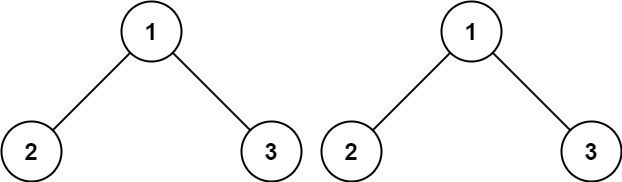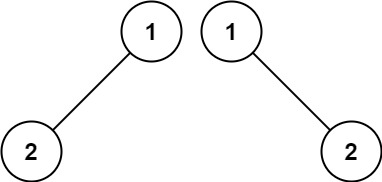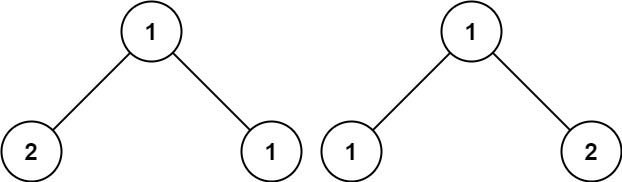# LeetCode: 100. Same Tree

## 题目¶

Given the roots of two binary trees p and q, write a function to check if they are the same or not.

Two binary trees are considered the same if they are structurally identical, and the nodes have the same value.

Example 1:```Input: p = [1,2,3], q = [1,2,3]
Output: true
```

Example 2:```Input: p = [1,2], q = [1,null,2]
Output: false
```

Example 3:```Input: p = [1,2,1], q = [1,1,2]
Output: false
```

Constraints:

• The number of nodes in both trees is in the range [0, 100].
• -104 <= Node.val <= 104

## 解法¶

```# Definition for a binary tree node.
# class TreeNode(object):
#     def __init__(self, val=0, left=None, right=None):
#         self.val = val
#         self.left = left
#         self.right = right
class Solution(object):
def isSameTree(self, p, q):
return self.is_same(p, q)

def is_same(self, a, b):
if a is None and b is None:
return True
if a is None or b is None:
return False
if a.val != b.val:
return False

return self.is_same(a.left, b.left) and self.is_same(a.right, b.right)
```